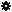﻿ Object Model: Edge.NeighborVertices

Edge.NeighborVertices

## Edge.NeighborVerticesv3.0

### Description

Returns a collection of Vertex objects for all vertex neighbors within a given distance. If the distance is 1, the 2 Vertex objects comprising the current Edge are returned.

### C# Syntax

 ```VertexCollection Edge.NeighborVertices( Int32 in_lDistance ); ```

### Scripting Syntax

 ```oReturn = Edge.NeighborVertices( [Distance] ); ```

### Parameters

Parameter Type Description
Distance Integer Distance is an integer value representing the degree of neighborhood (for example, degree=2 for an edge means its adjacent vertices plus the adjacent vertices of the adjacent vertices).

Default Value: 1

### Examples

#### VBScript Example

 ```set oCube = ActiveSceneRoot.AddGeometry( "Cube", "MeshSurface" ) set oGeometry = oCube.ActivePrimitive.Geometry for each Edge in oGeometry.Edges set oNeighbors = Edge.NeighborVertices( 1 ) str = "Vertex neighbors of edge( " & Edge.index & ") are :" for each n in oNeighbors str = str & " " & n.index next logmessage str next ```